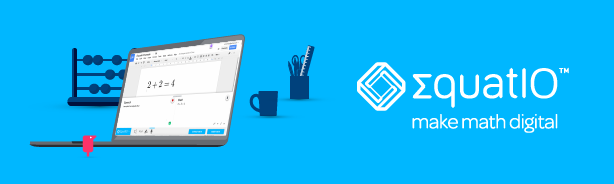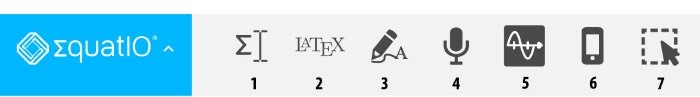# Equatio by TextHelp## What is EquatIO?

EquatIO is software from Texthelp that helps people create accessible digital math without complex coding or math languages to master. EquatIO runs on Mac and Windows, and from Google Chrome in the G-Suite apps. Miami University has limited seating for EquatIO. If you are interested in using this software, please contact the AccessMU Center at AccessMU@MiamiOH.edu.

## Challenges of Math Expressions

Images of math expressions are commonly used in documents or web pages. These can be difficult to interpret for students with learning disabilities like dyslexia or dyscalculia, and those who use assistive technology. EquatIO provides tools to create digital math expressions that are more accessible to all.## The EquatIO toolbar

1. The EquatIO editor allows you to type math expressions into the editor directly. The prediction feature makes entering common math and chemistry expressions quicker and more accurate.
2. EquatIO understands input in LaTeX, the typesetting and mark-up language that’s widely used for mathematical and scientific documents and publications. The LaTex editor allows people to enter code and EquatIO provides alternative outputs including MathML which is accessible to screen readers, and as spoken text which can be used as alternative text for math expressions inserted as images.
3. The handwriting recognition option in the EquatIO toolbar lets you write math expressions with a mouse or stylus (on touch devices). EquatIO converts the writing to digital math quickly and easily.
4. The speech input option lets you speak math. EquatIO converts it to a digital expression that is ready to insert into your document.
5. The graph editor leverages the power of the Desmos HTML5 graphing calculator to make math visual. Enter an expression and see it plotted instantly.
6. EquatIO mobile uses math optical character recognition (OCR), handwriting and speech input on your mobile device to insert math expressions into any document.
7. The EquatIO screenshot reader lets you turn any inaccessible equation on the web into accessible, editable math and automatically reads it aloud. This powerful feature supports UDL principles by providing an alternative modality to comprehend math. Once converted, you can also copy the MathML of the equation, which can then be pasted directly into the EquatIO equation editor for edits or insertion into your document.